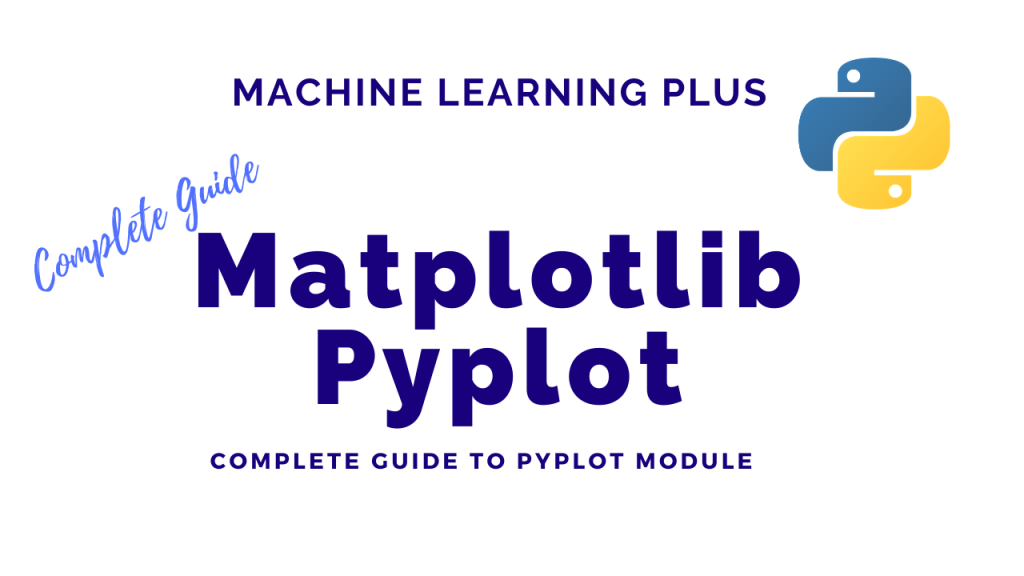## Matplotlib Pyplot – How to import matplotlib in Python and create different plots

The pyplot object is the main workhorse of matplotlib library. It is through pyplot that you can create the figure canvas, various types of plots, modify and decorate them. Contents Pyplot: Basic Overview General Functions in pyplot Line plot Scatter plot Pie chart Histogram 2D Histograms Bar plot Stacked Barplot Boxplot Stackplot Time series plotting …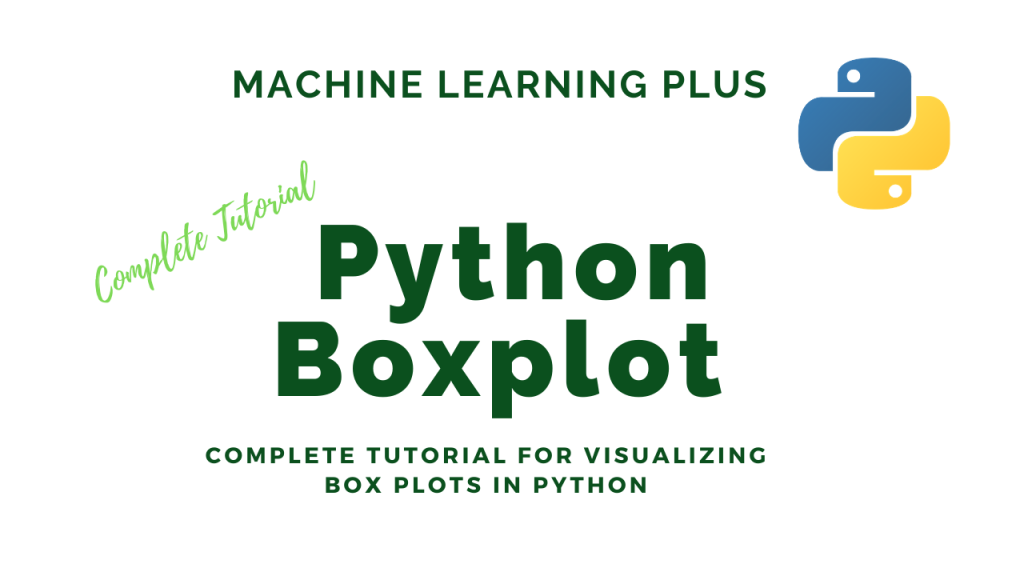## Python Boxplot – How to create and interpret boxplots (also find outliers and summarize distributions)

Boxplot is a chart that is used to visualize how a given data (variable) is distributed using quartiles. It shows the minimum, maximum, median, first quartile and third quartile in the data set. What is a boxplot? Box plot is method to graphically show the spread of a numerical variable through quartiles. From the below …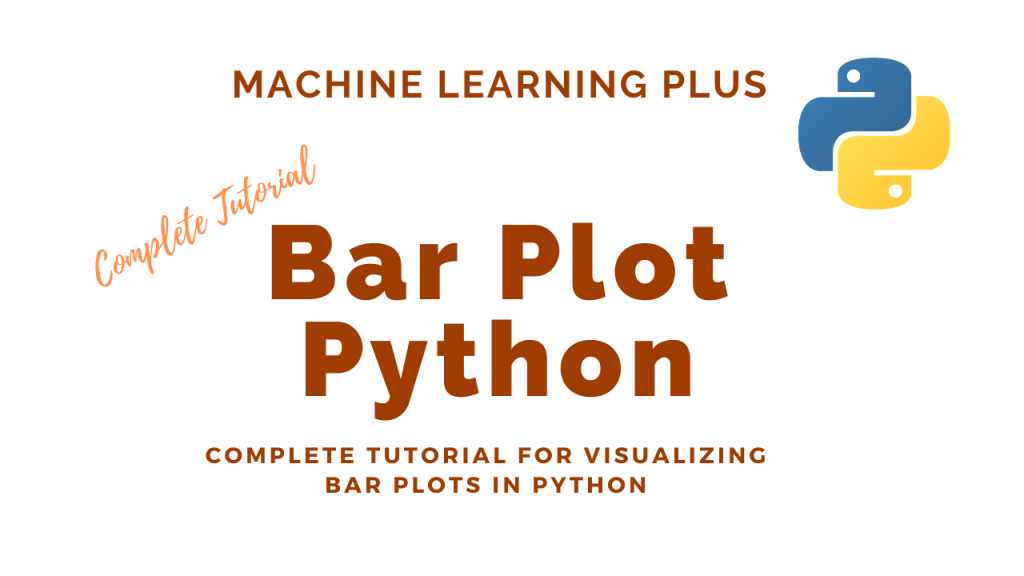## Bar Plot in Python – How to compare Groups visually

A bar plot shows catergorical data as rectangular bars with the height of bars proportional to the value they represent. It is often used to compare between values of different categories in the data. Content What is a barplot? Simple bar plot using matplotlib Horizontal barplot Changing color of a barplot Grouped and Stacked Barplots …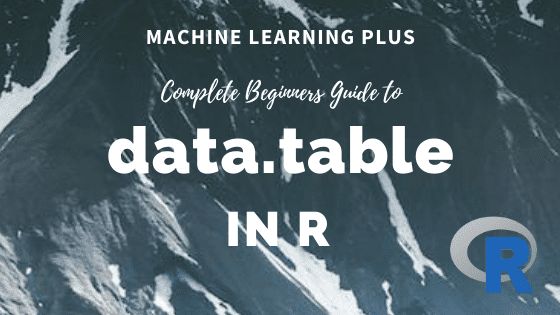## data.table in R – The Complete Beginners Guide

data.table is a package is used for working with tabular data in R. It provides the efficient data.table object which is a much improved version of the default data.frame. It is super fast and has intuitive and terse syntax. If you know R language and haven’t picked up the data.table package yet, then this tutorial …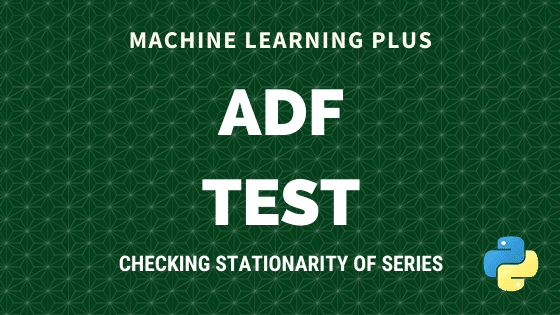Augmented Dickey Fuller test (ADF Test) is a common statistical test used to test whether a given Time series is stationary or not. It is one of the most commonly used statistical test when it comes to analyzing the stationary of a series. 1. Introduction In ARIMA time series forecasting, the first step is to …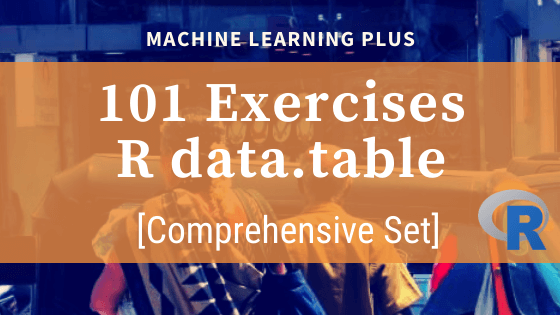## 101 R data.table Exercises

The data.table package in R is super fast when it comes to handling data. It has a syntax that reduces keystrokes while making R code easier to read. These set of exercises are designed to help you to oil your data brain through solving data manipulation exercises. Related post: 101 Python datatable Exercises (pydatatable) 101 …## What is P-Value? – Understanding the meaning, math and methods

P Value is a probability score that is used in statistical tests to establish the statistical significance of an observed effect. Though p-values are commonly used, the definition and meaning is often not very clear even to experienced Statisticians and Data Scientists. In this post I will attempt to explain the intuition behind p-value as …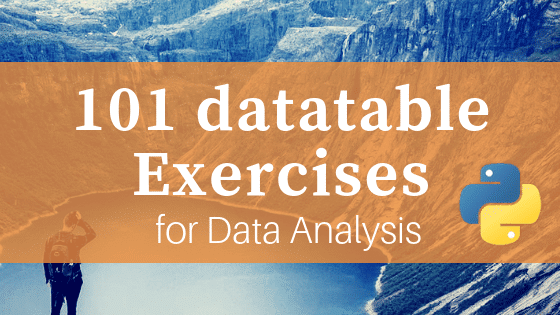## 101 Python datatable Exercises (pydatatable)

Python datatable is the newest package for data manipulation and analysis in Python. It carries the spirit of R’s data.table with similar syntax. It is super fast, much faster than pandas and has the ability to work with out-of-memory data. Looking at the performance it is on path to become a must-use package for data …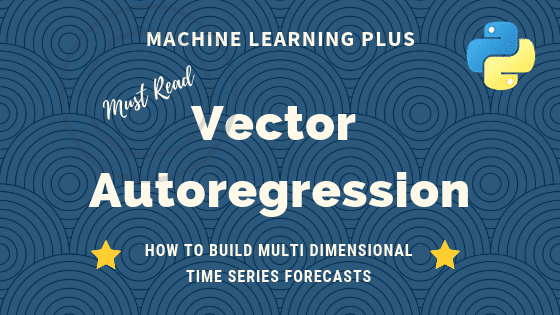## Vector Autoregression (VAR) – Comprehensive Guide with Examples in Python

Vector Autoregression (VAR) is a forecasting algorithm that can be used when two or more time series influence each other. That is, the relationship between the time series involved is bi-directional. In this post, we will see the concepts, intuition behind VAR models and see a comprehensive and correct method to train and forecast VAR …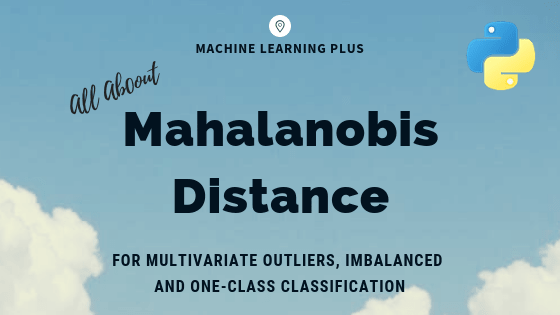## Mahalanobis Distance – Understanding the math with examples (python)

Mahalanobis distance is an effective multivariate distance metric that measures the distance between a point and a distribution. It is an extremely useful metric having, excellent applications in multivariate anomaly detection, classification on highly imbalanced datasets and one-class classification. This post explains the intuition and the math with practical examples on three machine learning use …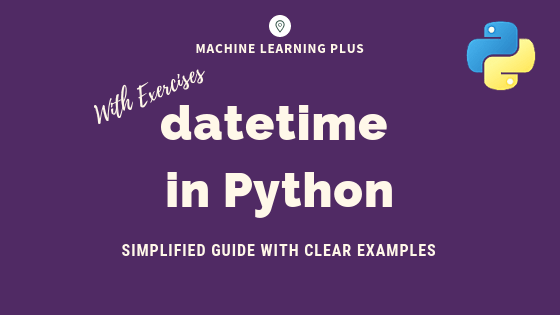## datetime in Python – Simplified Guide with Clear Examples

datetime is the standard module for working with dates in python. It provides 4 main objects for date and time operations: datetime, date, time and timedelta. In this post you will learn how to do all sorts of operations with these objects and solve date-time related practice problems (easy to hard) in Python. datetime in …## Principal Component Analysis – How PCA algorithms works, the concept, math and implementation

Principal Components Analysis (PCA) is an algorithm to transform the columns of a dataset into a new set of features called Principal Components. By doing this, a large chunk of the information across the full dataset is effectively compressed in fewer feature columns. This enables dimensionality reduction and ability to visualize the separation of classes …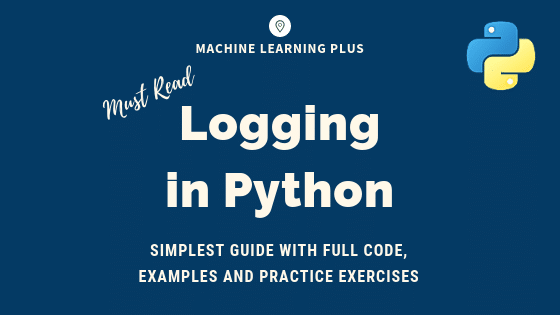## Python Logging – Simplest Guide with Full Code and Examples

The logging module lets you track events when your code runs so that when the code crashes you can check the logs and identify what caused it. Log messages have a built-in hierarchy – starting from debugging, informational, warnings, error and critical messages. You can include traceback information as well. It is designed for small …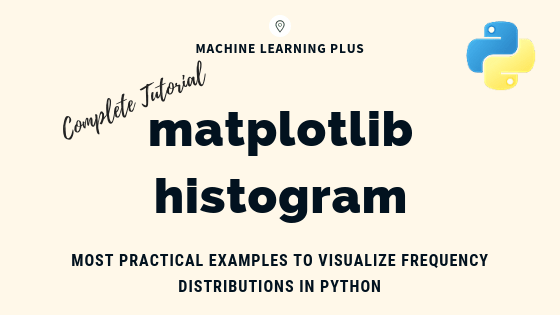## Matplotlib Histogram – How to Visualize Distributions in Python

Matplotlib histogram is used to visualize the frequency distribution of numeric array by splitting it to small equal-sized bins. In this article, we explore practical techniques that are extremely useful in your initial data analysis and plotting. Content What is a histogram? How to plot a basic histogram in python? Histogram grouped by categories in …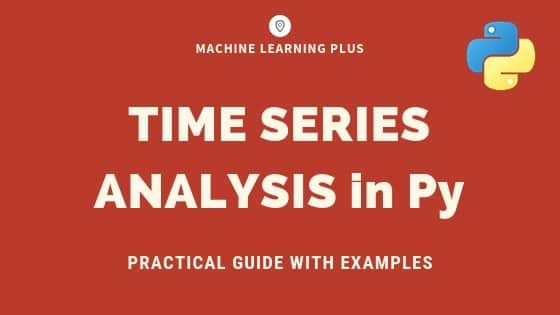## Time Series Analysis in Python – A Comprehensive Guide with Examples

Time series is a sequence of observations recorded at regular time intervals. This guide walks you through the process of analyzing the characteristics of a given time series in python. Time Series Analysis in Python – A Comprehensive Guide. Photo by Daniel Ferrandiz. Contents What is a Time Series? How to import Time Series in …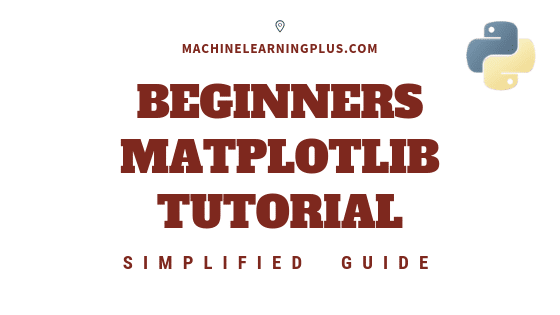## Matplotlib Tutorial – A Complete Guide to Python Plot with Examples

Until next time Until next time This tutorial explains matplotlib’s way of making plots in simplified parts so you gain the knowledge and a clear understanding of how to build and modify full featured matplotlib plots. 1. Introduction Matplotlib is the most popular plotting library in python. Using matplotlib, you can create pretty much any …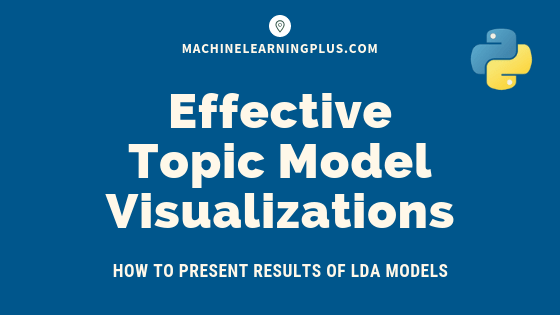## Topic modeling visualization – How to present the results of LDA models?

In this post, we discuss techniques to visualize the output and results from topic model (LDA) based on the gensim package. Topic modeling visualization – How to present the results of LDA models? Contents Introduction Import NewsGroups Dataset Tokenize Sentences and Clean Build the Bigram, Trigram Models and Lemmatize Build the Topic Model Presenting the …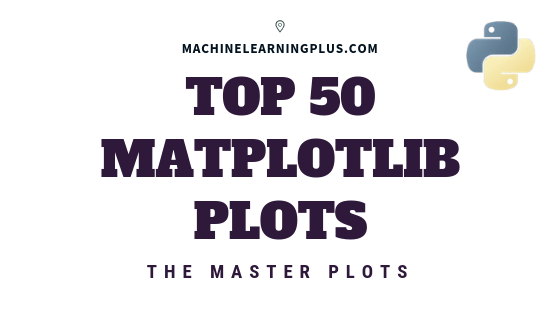## Top 50 matplotlib Visualizations – The Master Plots (with full python code)

A compilation of the Top 50 matplotlib plots most useful in data analysis and visualization. This list lets you choose what visualization to show for what situation using python’s matplotlib and seaborn library. Introduction The charts are grouped based on the 7 different purposes of your visualization objective. For example, if you want to picturize …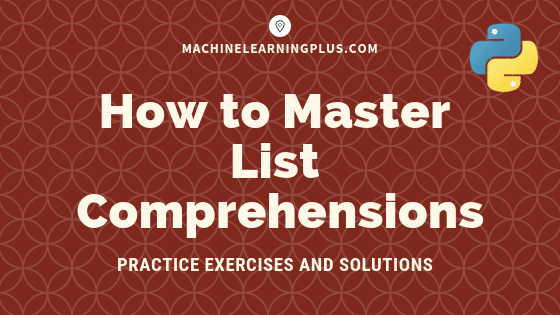## List Comprehensions in Python – My Simplified Guide

List comprehensions is a pythonic way of expressing a ‘For Loop’ that appends to a list in a single line of code. It is an intuitive, easy-to-read and a very convenient way of creating lists. This is a beginner friendly post for those who know how to write for-loops in python but don’t quite understand …Course Preview

## Machine Learning A-Z™: Hands-On Python & R In Data Science

### Free Sample Videos:#### Machine Learning A-Z™: Hands-On Python & R In Data Science#### Machine Learning A-Z™: Hands-On Python & R In Data Science#### Machine Learning A-Z™: Hands-On Python & R In Data Science#### Machine Learning A-Z™: Hands-On Python & R In Data Science